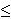## Eigenvalue-nonhamiltonian graphs

Let G be a cubic graph of order n, and let 0 = t1t2...tn be its Laplace eigenvalues. The following result was proved in .

Theorem 1: If there is an index k such that either
(i)  tk > 4 - 2 cos(2[k/2] / n), where [.] denotes the integer part, or
(ii) n / 2 < kn and tk < 4 - 2 cos(2[(5n + 2 - 2k)/4] / n),
then G does not contain a hamilton cycle.

According to this result, a cubic graph is called eigenvalue-nonhamiltonian if its Laplace eigenvalues satisfy (i) or (ii). It can be verified that the Petersen graph P is eigenvalue-nonhamiltonian. This result therefore provides an algebraic proof that P has no hamilton cycle. It would be interesting to find a 3-connected cubic eigenvalue-nonhamiltonian graph distinct from the Petersen graph. Is there an infinite family of such graphs? In the past, I have tried to show that some Cayley graphs of PSL groups are eigenvalue-nonhamiltonian. Having no success, I am posing the following

Conjecture: If G is a 3-connected cubic eigenvalue-nonhamiltonian graph, then it is isomorphic to the Petersen graph.

On the other hand, it would be interesting to find other algebraic criteria forcing nonhamiltonicity of graphs.

References:

 B. Mohar, A domain monotonicity theorem for graphs and hamiltonicity, Discr. Appl. Math. 36 (1992) 169-177.